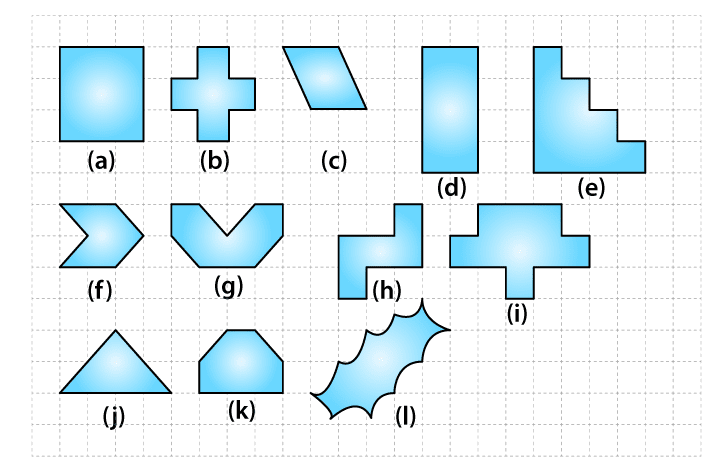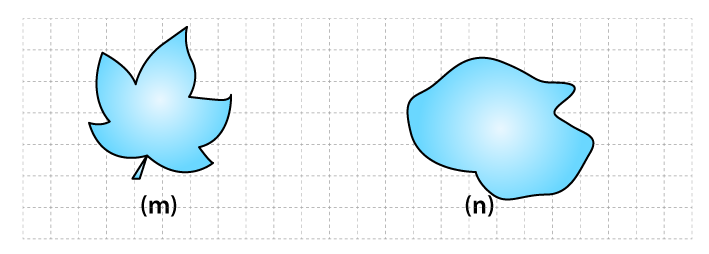## Access NCERT Solutions for Class 6 Maths Chapter 10: Mensuration Exercise 10.2

### Access NCERT Solutions for Class 6 Maths Chapter 10: Mensuration Exercise 10.2

1. Find the areas of the following figures by counting square:(a) The figure contains only 9 fully filled squares. Hence, the area of this figure will be 9 square units.

(b) The figure contains only 5 fully filled squares. Hence, the area of this figure will be 5 square units.

(c) The figure contains 2 fully filled squares and 4 half filled squares. Hence, the area of this figure will be 4 square units.

(d) The figure contains only 8 fully filled squares. Hence, the area of this figure will be 8 square units.

(e) The figure contains only 10 fully filled squares. Hence, the area of this figure will be 10 square units.

(f) The figure contains only 2 fully filled squares and 4 half filled squares. Hence, the area of this figure will be 4 square units.

(g) The figure contains 4 fully filled squares and 4 half filled squares. Hence, the area of this figure will be 6 square units.

(h) The figure contains 5 fully filled squares. Hence, the area of this figure will be 5 square units.

(i) The figure contains 9 fully filled squares. Hence, the area of this figure will be 9 square units.

(j) The figure contains 2 fully filled squares and 4 half filled squares. Hence, the area of this figure will be 4 square units.

(k) The figure contains 4 fully filled squares and 2 half filled squares. Hence, the area of this figure will be 5 square units.

(l) From the given figure, we observe

 Covered Area Number Area estimate (square units) Fully filled squares 2 2 Half filled squares – – More than half filled squares 6 6 Less than half filled squares 6 0

Therefore total area = 2 + 6

= 8 square units.

(m) From the given figure, we observe

 Covered Area Number Area estimate (square units) Fully filled squares 5 5 Half filled squares – – More than half filled squares 9 9 Less than half filled squares 12 0

Therefore total area = 5 + 9

= 14 square units

(n) From the given figure, we observe

 Covered Area Number Area estimate (square units) Fully filled squares 8 8 Half filled squares – – More than half filled squares 10 10 Less than half filled squares 9 0

Therefore total area = 8 + 10 = 18 square units